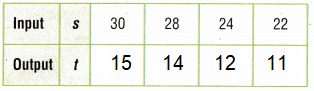# Texas Go Math Grade 4 Lesson 12.2 Answer Key Find a Rule

Refer to our Texas Go Math Grade 4 Answer Key Pdf to score good marks in the exams. Test yourself by practicing the problems from Texas Go Math Grade 4 Lesson 12.2 Answer Key Find a Rule.

## Texas Go Math Grade 4 Lesson 12.2 Answer Key Find a Rule

Essential Question

How can you write the rule for a function?
A function is a rule which relates the values of one variable quantity to the values of another variable quantity, and does so in such a way that the value of the second variable quantity is uniquely determined by the value of the first variable quantity.

Unlock the Problem

A server at a restaurant is setting up tables for a large group. One table has 4 chairs. Two tables have 8 chairs. Three tables have 12 chairs. Four tables have 16 chairs, and so on. How many chairs are at 5 tables?

• Underline the information you will use.
• Circle the numbers of chairs.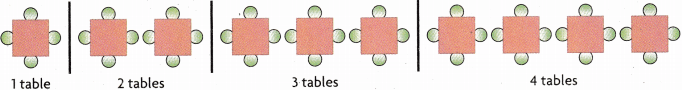You can use an input/output table to show a pattern. A pattern is called a function when one quantity depends on the other. The number of chairs that can be used depends on the number of tables used. You can write a rule to describe the relationship between the inputs and outputs of a function.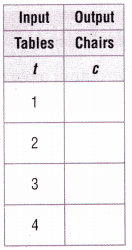Use a table to write a rule.

ERROR Alert: A rule must work for each pair of numbers in the function table. Be sure to test your rule for each pair of numbers.

STEP 1 Complete the input/output table.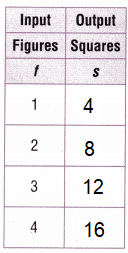Explanation:
Rule: Multiply by 4
Input t = 1, 2, 3, 4
Output s = t x 4

STEP 2 Describe the relationship between tables and chairs.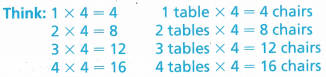The number of chairs is __________ times the number of tables.
s = t x 4
The number of chairs is 4 times the number of tables.
Explanation:
Rule: Multiply by 4
Input t = 1, 2, 3, 4
Output s = t x 4

STEP 3 Find a rule. Use an expression to write your rule.
Think: Use t for the number of tables.
Rule: The number of chairs is __________ × __________.
Rule: The number of chairs is t × 4Explanation:
Rule: Multiply by 4
Input t = 1, 2, 3, 4
Output s = t x 4

STEP 4 Use the rule to find the number of chairs at 5 tables.
The number of chairs at 5 tables is 5 × __________.
So, there are __________ chairs at 5 tables.
The number of chairs at 5 tables is 5 × 4
So, there are 20 chairs at 5 tables.
Explanation:
Rule: Multiply by 4
Input t = 5
Output s = t x 4
s = 5 x 4 = 20

Math Talk

Mathematical Processes
Explain how you can use the rule to find the number of chairs at 8 tables.
The number of chairs at 8 tables is 4 × 8
So, there are 32 chairs at 8 tables.Examples

(A) Find a rule. Use your rule to write an expression.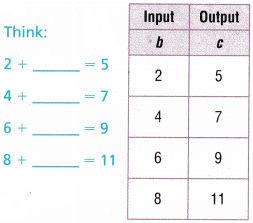The output is __________ more than the input.
Use __________ for the input.
Rule: The output is __________ + __________.
The output is 3 more than the input.
Use b for the input.
Rule: The output is b + 3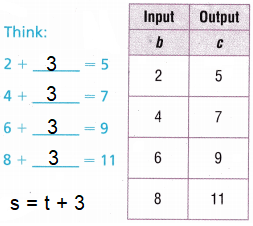Explanation:
Input b = 2, 4, 6, 8
Output c = b + 3

(B) Use the rule to complete the input/output table.
Rule: The output is n ÷ 2.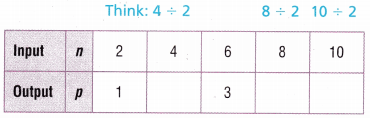Rule: The output is n ÷ 2.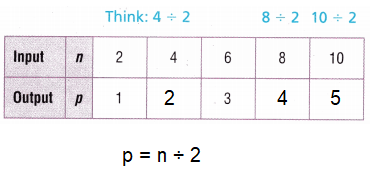Explanation:
Rule: The output is n ÷ 2.
Input n = 2, 4, 6, 8, 10
Output p = n ÷ 2

Share and Show

Question 1.
Use the input/output table to show the pattern. Find a rule.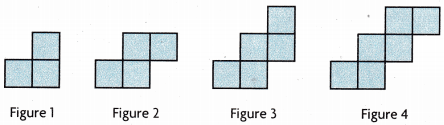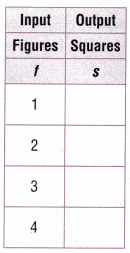Rule: The number of square is __________ + ___________ .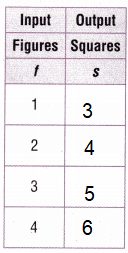Rule: The number of square is t + 2
Explanation:
Input t = 1, 2, 3, 4
Output s = t + 2
s = t + 2
s = 1 + 2 = 3
s = 2 + 2 = 4
s = 3 + 2 = 5
s = 4 + 2 = 6

Use the rule to complete the input/output table.

Rule: The output is n – 5.Explanation:
Rule: The output is n – 5.
n = 10, 20, 30 ,40
p = n -5
p = 10 – 5 = 5
p = 20 – 5 = 15
p = 30 – 5 = 25
p = 40 – 5 = 35

Math Talk

Mathematical Processes
Explain how you can find a rule.
A function is a rule which relates the values of one variable quantity to the values of another variable quantity, and does so in such a way that the value of the second variable quantity is uniquely determined by the value of the first variable quantity.

Find a rule. Use your rule to write an expression.

Question 3.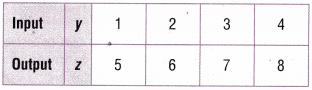Rule: _________________
Rule: The output is y + 4
Explanation:
output y = 1, 2, 3, 4
input z = y + 4
z = 1 + 4 = 5
z = 2 + 4 = 6
z = 3 + 4 = 7
z = 4 + 4 = 8

Question 4.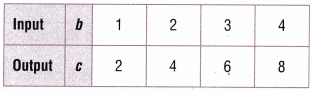Rule: _________________
Rule: The output is b x 2
Explanation:
Rule: Multiply by 3 to the first term
Output b = 1, 2, 3, 4
Input c = b x 2
c = 1 x 2 = 2
c = 2 x 2 = 4
c = 3 x 2 = 6
c = 4 x 2 = 8

Problem Solving

Question 5.
Record Use the food pyramid to complete the input/output table below. Find a rule that tells how many cups of milk a child should drink in d days.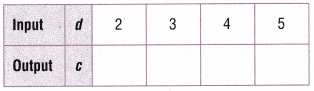c = d x 3
cups of milk a child should drink in d days.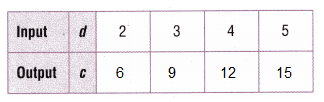Explanation:
Rule: Multiply by 3 to the d term
Output c = 2, 3, 4,5
Input c = d x 3
c = 2 x 3 = 6
c = 3 x 3 = 9
c = 4 x 3 = 12
c = 5 x 3 = 15

4th Grade Lesson 12.2 Input and Output Tables Question 6.
One gallon of water is equivalent to 4 quarts of water. You can write a rule to show this.

Let g represent the number of gallons of water, the input, and let q represent the total number of quarts of water, the output.

Rule: The number of quarts is g × 4.
Use the rule to complete the input/output table.Rule: Multiply by 4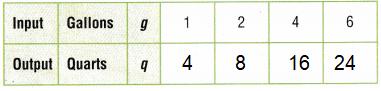Explanation:
Rule: Multiply by 4
g = 1,2, 3, 4, 6
q = g x 4
q = 1 x 4 = 4
q = 2 x 4 = 8
q = 3 x 4 = 12
q = 4 x 4 = 16

Question 7.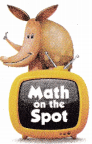H.O.T. Analyze One gallon of water equals 4 quarts of water and 1 quart of water equals 2 pints of water. How many pints of water equal 1 gallon of water? Explain.
1 gallon of water = 8 points of water
Explanation:
One gallon = 4 quarts
1 quarts = 2 points
So, 1 gallon of water = 8 points of water.

Question 8.
H.O.T. Look back at Problem 6. What if there are 36 quarts of water?
How many gallons of water are there? ____________
9 gallons of water are there.
Explanation: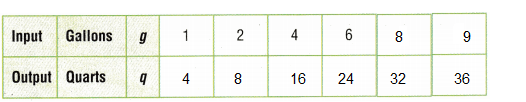g = 1,2, 3, 4, 6, 8, 9
q = g x 4
q = 9 x 4 = 36

Question 9.
Tanya is waiting in line at a skate park There are 4 people in front of her. The input/output table shows the relationship between the number of people (p) in line and the length of time (t) she will wait in line. Which could be a rule for the input/output table?(A) The output is p + 3.
(B) The output is p × 4.
(C) The output is t – 3.
(D) The output is p× 2.
Option(B)
Explanation:
Input p = 1,2, 3, 4, 6
Output t = p x 4
t = 1 x 4 = 4
t = 2 x 4 = 8
t = 3 x 4 = 12
t = 4 x 4 = 16

Question 10.
Use the rule to find the missing number.
What is the missing number?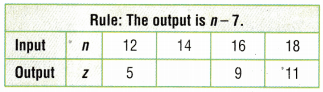(A) 21
(B) 8
(C) 7
(D) 2
Option(C)
Explanation:
Rule: The output n – 7
n = 12, 14, 16, 18
z = n – 7
z = 12 – 7 = 5
z = 14 – 7 = 7
z = 16 – 7 = 9
z = 18 – 7 = 11

Multi-Step In a photo album, one page holds 4 photographs. Two pages hold 8 photographs. Three pages hold 12 photographs. Four pages hold 16 photographs, and so on. How many photographs do 5 pages hold?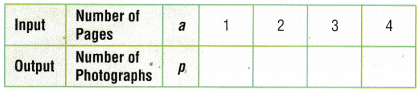(A) 5
(B) 18
(C) 24
(D) 20
Option(D)
Explanation:
Rule: Multiply by 4
Input a = 1, 2, 3, 4
Output p = a x 4
p = 1 x 4 = 4
p = 2 x 4 = 8
p = 3 x 4 = 12
p = 4 x 4 = 16

TEXAS Test Prep

Question 12.
Maggie runs the same distance every week. She made the input/output table to show how many miles (m) she will run in w weeks. Which rule Output works for the input/output table?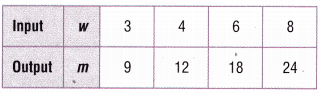(A) The output is w + 6.
(B) The output is w ÷ 2.
(C) The output is w × 3.
(D) The output is w ÷ 3.
Option(C)
Explanation:
Rule: The output is w × 3.
w = 3, 4, 6, 8
Input m = w x 3
m = 3 x 3 = 9
m = 4 x 3 = 12
m = 6 x 3 = 18
m = 8 x 3 = 24

### Texas Go Math Grade 4 Lesson 12.2 Homework and Practice Answer Key

Use the rule to complete the input/output table.

Question 1.
Rule: The output is n + 3.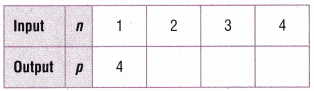Rule: The output is n + 3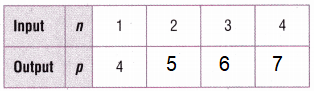Explanation:
Rule: The output is n + 3.
n = 1, 2, 3, 4
p = n + 3
p = 1 + 3 = 4
p = 2 + 3 = 5
p = 3 + 3 = 6
p = 4 + 3 = 7

Question 2.
Rule: The output is n × 5.Rule: The output is n × 5.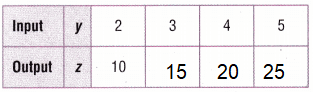Explanation:
Rule: The output is n × 5.
n = 2, 3, 4, 5
z = n x 5
z = 2 x 5 = 10
z = 3 x 5 = 15
z = 4 x 5 = 20
z = 5 x 5 = 25

Question 3.
Rule: The output is f – 4.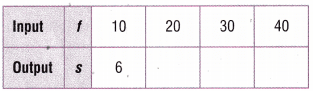Rule: The output is f – 4.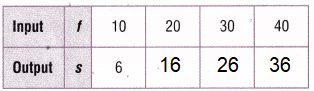Explanation:
Rule: The output is f – 4.
f = 10, 20, 30, 40
s = f – 4
s = 10 – 4 = 6
s = 20 – 4 = 16
s = 30 – 4 = 26
s 40 4 = 36

Question 4.
Rule: The output is x ÷ 10.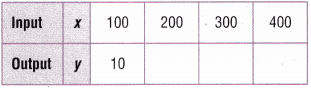Rule: The output is x ÷ 10.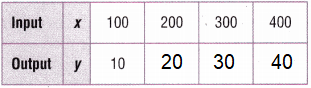Explanation:
Rule: The output is x ÷ 10.
Output x = 100, 200, 300, 400
Input y = x ÷ 10
y = 100 ÷ 10 = 10
y = 200 ÷ 10 = 20
y = 300 ÷ 10 = 30
y = 400 ÷ 10 = 40

Find a rule. Use your rule to write an expression.

Question 5.Rule: _________________
Rule: The Output is y + 5
Explanation:
Output y = 1, 2, 3, 4
Input z = y + 5
z = 1 + 5 = 6
z = 2 + 5 = 7
z = 3 + 5 = 8
Z = 4 + 5 = 9

Question 6.Rule: __________________
Rule: The output is b x 3
Explanation:
Rule: Multiply by 3 to the first term
Output b = 1, 2, 3, 4
Input c = b x 3
c = 1 x 3 = 3
c = 2 x 3 = 6
c = 3 x 3 = 9
c = 4 x 3 = 12

Problem Solving

Question 7.
One gallon of water is equal to 8 pints of water. Find a rule that tells how many pints of water are in g gallons.
Rule: __________________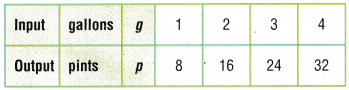Rule: The output is g x 8
Explanation:
Rule: Multiply by 8 to the first term
output g = 1, 2, 3, 4
input p = g x 8
p = 1 x 8 =8
p = 2 x 8 =16
p = 3 x 8 =24
p = 4 x 8 =32

Question 8.
One quart of water is equal to 4 cups of water. Find a rule that tells how many cups of water are in q quarts.
Rule: __________________Rule: The output is q x 4
Explanation:
Rule: Multiply by 4 to the first term
output q = 1, 2, 3, 4
input p = g x 8
c = 1 x 4 =4
c = 2 x 4 =86
c = 3 x 4 =12
c = 4 x 4 =16

Lesson Check

Question 9.
Use the rule to find the missing number, What is the missing number?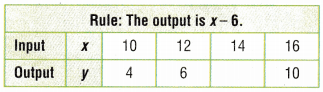(A) 20
(B) 2
(C) 8
(D) 5
Option(C)
Explanation:
Rule: The output is x -6
output x = 10, 12, 14, 16
input y = x – 6
10 – 6 = 4
12 – 6 = 6
14 – 6 = 8
16 – 6 = 10

Question 10.
Which rule could be used for the table?(A) The output is n + 10.
(B) The output is n × 2.
(C) The output is n – 2.
(D) The output is n – 10.
Option(B)
Explanation:
Rule: The output is n x 10
output n = 10, 15, 20, 25
input p = n x 2
p = 10 x 2 = 20
p = 15 x 2 = 30
p = 20 x 2 = 40
p = 25 x 2 = 50

Question 11.
Nikki is making necklaces. The input/ output table shows the relationship between the number of necklaces (n) and the number of heads (b) used.Which could be a rule for the table?
(A) The output is n × 8.
(B) The output is n + 14.
(C) The output is n + 8.
(D) The output is n – 14.
Option(A)
Explanation:
Rule: The output is n x 8
output n = 2, 4, 6, 8
input p = n x 8
b = 2 x 8 = 16
b = 4 x 8 = 32
b = 6 x 8 = 48
b = 8 x 8 = 64

Ken works the same number of hours each week. He made the input/output table to show how many hours (h) he will work in d days.Which could be a rule for the table?
(A) The output is d – 30.
(B) The output is d ÷ 7.
(C) The output is d × 7.
(D) The output is d + 30.
Option(C)
Explanation:
Rule: The output is d x 7
output n = 2, 4, 6, 8
input h = d x 8
h = 5 x 7 = 35
h = 6 x 7 = 42
h = 7 x 7 = 49
h = 8 x 7 = 56

Question 13.
Multi-Step A baker packs cupcakes in boxes to sell. One box holds 6 cupcakes. Two boxes hold 12 cupcakes, and so on. How many cupcakes (c) will the baker need for 4 boxes (b)?(A) 6
(B) 24
(C) 18
(D) 12
Option(B)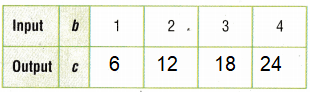Explanation:
Rule: The output is b x 2
output b = 1, 2, 3, 4
input c = n x 6
c = 1 x 6 = 6
c = 2 x 6 = 12
c = 3 x 6 = 18
c = 4 x 6 = 24

Question 14.
Multi-Step Students in a gym class will be divided into 2 teams to play games. For 30 students there will be 15 on each team. For 28 students, there will be 14 on each team, and so on. How many students (t) will be on each team if there are 22 students (s)?(A) 11
(B) 12
(C) 10
(D) 8